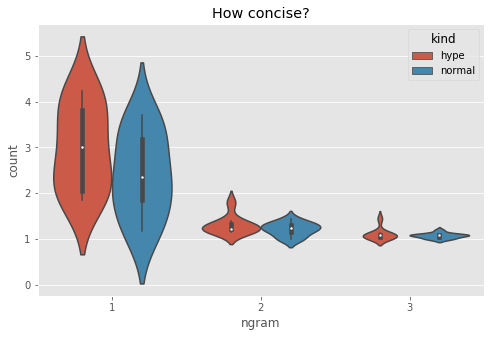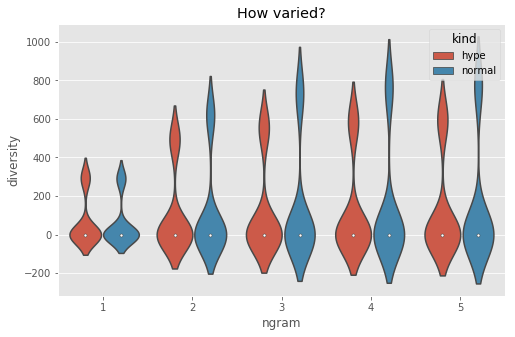30 March 2020 👏

# Signal & Noise

``````How to learn when the topic is drowned out by hype
``````

This is the code accompanying the talk Signal&Noise

```%pylab inline
plt.style.use('ggplot')

from collections import Counter, defaultdict
import pandas as pd
import seaborn as sns
import requests
from bs4 import BeautifulSoup
from urllib.parse import urlparse, parse_qs, urlencode
from newspaper import Article
import spacy

def search(q):
"""
We'll search google for the string provided and then return the content of the page as plain text.
"""
soup = BeautifulSoup(r.text, 'lxml')

links = [i for i in soup.find_all('a')]

articles = []
try:
a.parse()
except:
pass
else:
if a.text.strip() != '':
articles.append(a.text)
return articles```
```Populating the interactive namespace from numpy and matplotlib
```

Let's search for two topics. One that is clearly in the hype cycle and another that's the workhorse of computer science but is not being hyped about.

```ml = search('machine learning basics tutorial')
cpu = search('cpu scheduling basics tutorial')```

Let's measure n grams on the articles returned by the search. We'll consider up to 6-grams

```N = 6
texts = [[i for i in [w.text.lower().strip() for w in nlp.tokenizer(t) if not w.is_punct] if i != ''] for t in texts]
rows = []
for n in range(max_n):
grams = [list(zip(*[t[i:] for i in range(n)])) for t in texts]
for index, gr in enumerate(grams):
for key, val in Counter(gr).items():
rows.append([index, n, key, val])
df = pd.DataFrame(rows, columns=['doc', 'ngram', 'tpl', 'count'])
return df

Of these two topics, which one has more words but fewer concepts? A larger number of 1 grams and higher 4-6 grams would mean that people are typing a lot of stuff. The articles require more explanation?

```mldf['kind'] = 'hype'
cpudf['kind'] = 'standard'
df = pd.DataFrame([[count, n, kind]
for kind, df in [('hype', mldf), ('normal', cpudf)]
for n in range(4)
for count in df.loc[df.ngram == n].groupby('doc')['count'].mean()])
df.columns = ['count', 'ngram', 'kind']
plt.figure(figsize=(8, 5))
sns.violinplot(x='ngram', y='count', hue='kind', data=df)
plt.title('How concise?')```
`Text(0.5,1,'How concise?')`Let's see how different each article is from the others?

```mldf['kind'] = 'hype'
cpudf['kind'] = 'standard'

def variety(df):
multisets = defaultdict(dict)
docids = set(df.doc.values)
for ngram in sorted(df.ngram.unique()):
part = df.loc[df.ngram == ngram]
for docid in docids:
part = part.loc[part.doc == docid]
for _, row in part.iterrows():
multisets[docid, ngram][row.tpl] = 1
rows = []
for ngram in df.ngram.unique():
for this in docids:
for other in docids - set([this]):
a = set(multisets[this, ngram])
b = set(multisets[other, ngram])
count = len(a-b) + len(b-a)
rows.append((ngram, count))
return pd.DataFrame(rows, columns=['ngram', 'diversity'])

mldfv = variety(mldf)
mldfv['kind'] = 'hype'
cpudfv = variety(cpudf)
cpudfv['kind'] = 'normal'
plt.figure(figsize=(8, 5))
sns.violinplot(x='ngram', y='diversity', data=pd.concat([mldfv, cpudfv]), hue='kind')
plt.title('How varied?')```
`Text(0.5,1,'How varied?')`